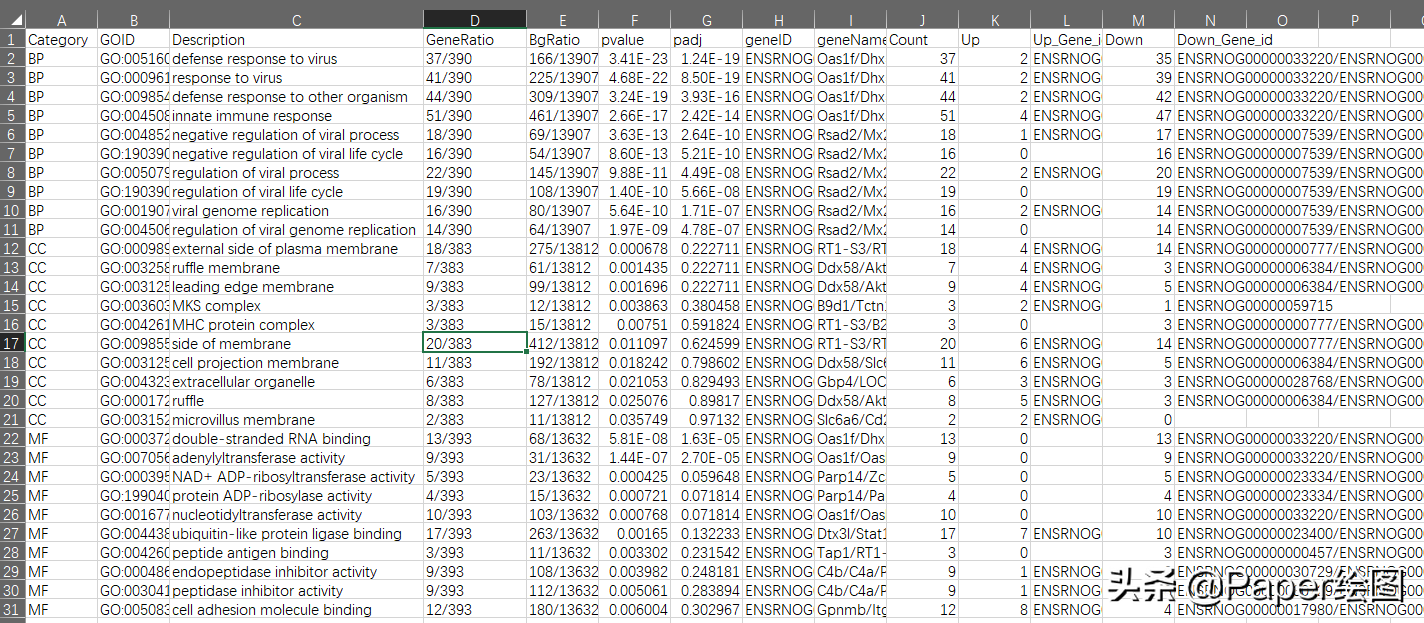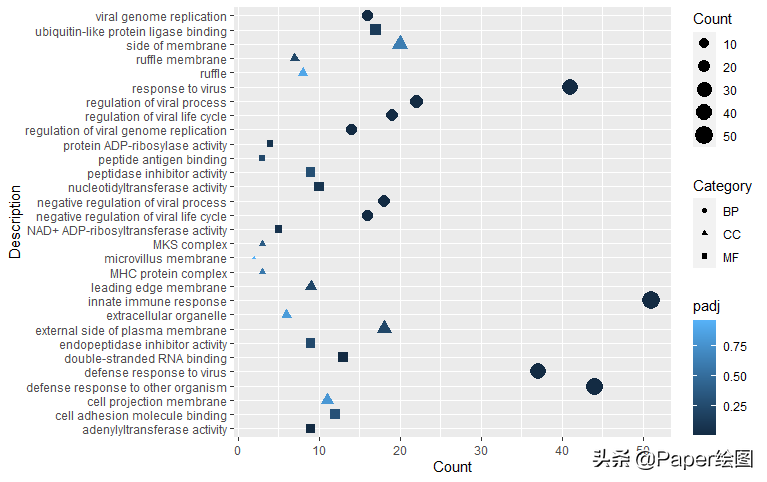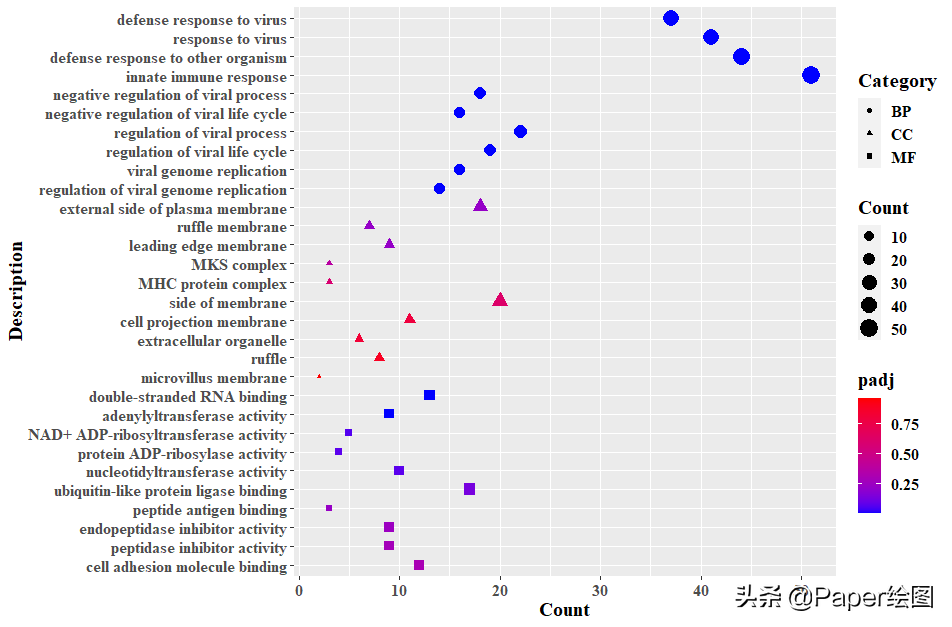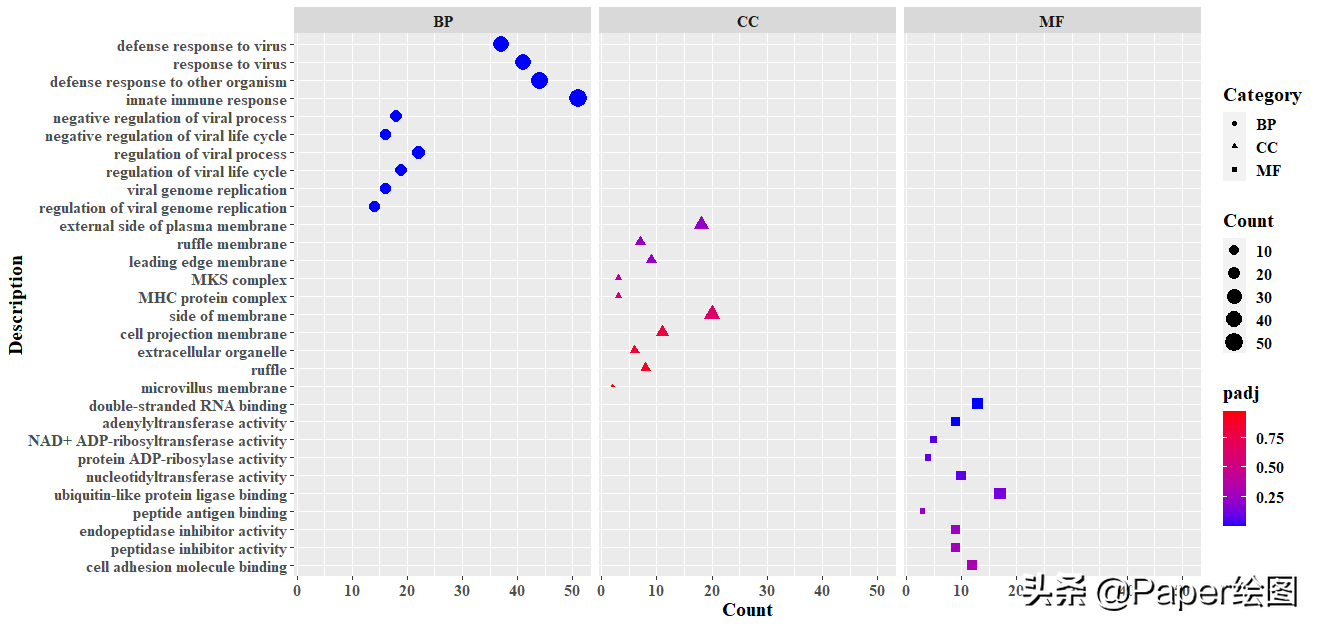# 软件介绍

[软件1]：R （Version 4.1.2）

[软件2]：RStudio（Version 1.4.1106）

[数据]：后台回复：气泡图数据，即可获得示例数据

# 教程讲解

1.加载需要的R包

``library(openxlsx)library(tidyverse)``

2.数据介绍，就是一般的GO富集分析结果，每一列代表一种类型的数据

• Category包括三个变量，分别是BP，CC，MF，接触过组学的同学都懂
• GOID就是GO术语的ID
• 其他列看第一列就明白意思了3.读取数据

``data <- read.xlsx("GO_气泡图.xlsx")``

4.使用ggplot2进行作图

• 将Category映射到shape，用不同的形状表示不同的生物过程
• 将Count映射到size，更加明显看到节点的大小
``ggplot(data,aes(Count,Description,                shape=Category,                colour=padj,                size=Count))+  geom_point()``5.进一步的对图形进行美化

• 使用windowsFonts函数提取系统字体
• data\$Description 这个函数的命名，完全是因为，在绘图过程中，y坐标上的术语按照字母顺序排列了，而我们需要让他按照原始文件的顺序排列
• 可能你么有发现，图上的Description与数据中的顺序刚好相反，因此使用scale_y_discrete()来进行调节
• guides()函数是用来调整图例的，这里的意思就是将Category放在第一
``data\$Description <- factor(data\$Description,levels = unique(data\$Description))windowsFonts(A=windowsFont("Times New Roman"))ggplot(data,aes(Count,Description,                shape=Category,                colour=padj,                size=Count))+  geom_point()+  theme(text = element_text(family = "A",size=15,face = "bold"))+  scale_color_gradient(low = "blue",high="red")+  scale_y_discrete(limits=rev(data\$Description))+  guides(shape=guide_legend(order = 1))``6.你也可以给他加一个分面

``ggplot(data,aes(Count,Description,                shape=Category,                colour=padj,                size=Count))+  geom_point()+  theme(text = element_text(family = "A",size=15,face = "bold"))+  scale_color_gradient(low = "blue",high="red")+  scale_y_discrete(limits=rev(data\$Description))+  guides(shape=guide_legend(order = 1))+  facet_grid(.~Category)``6.高级版气泡图，赶紧来学习一下吧！